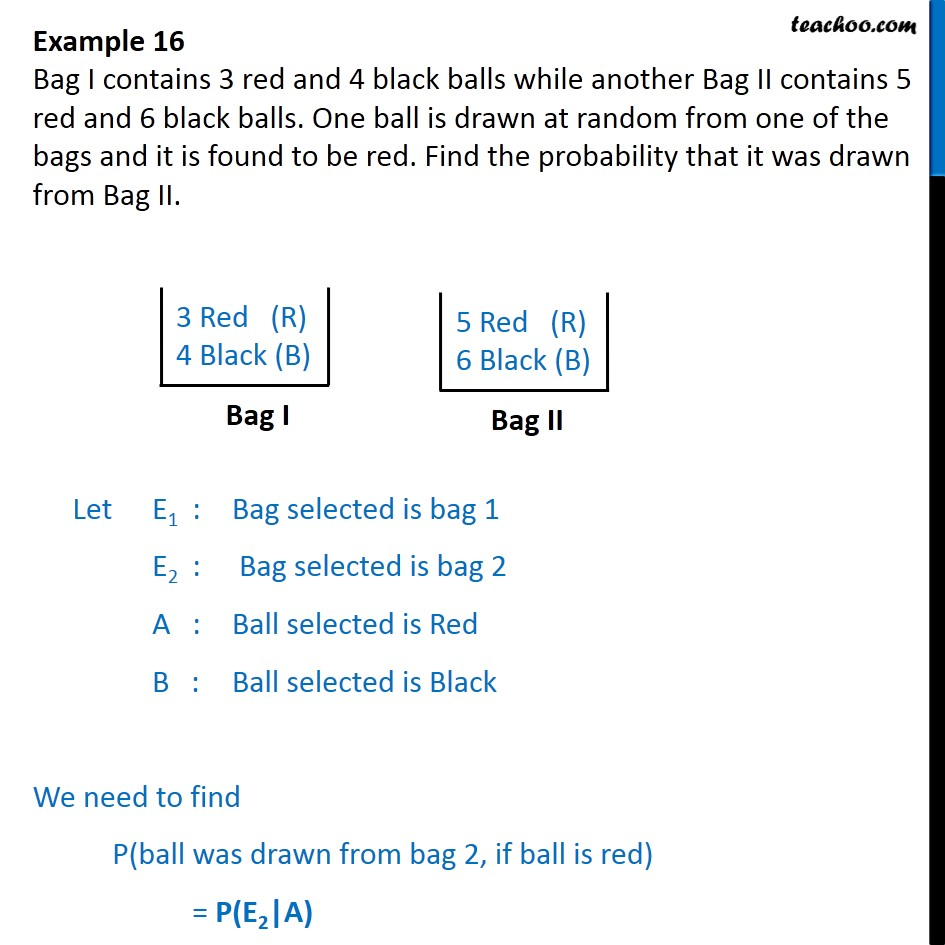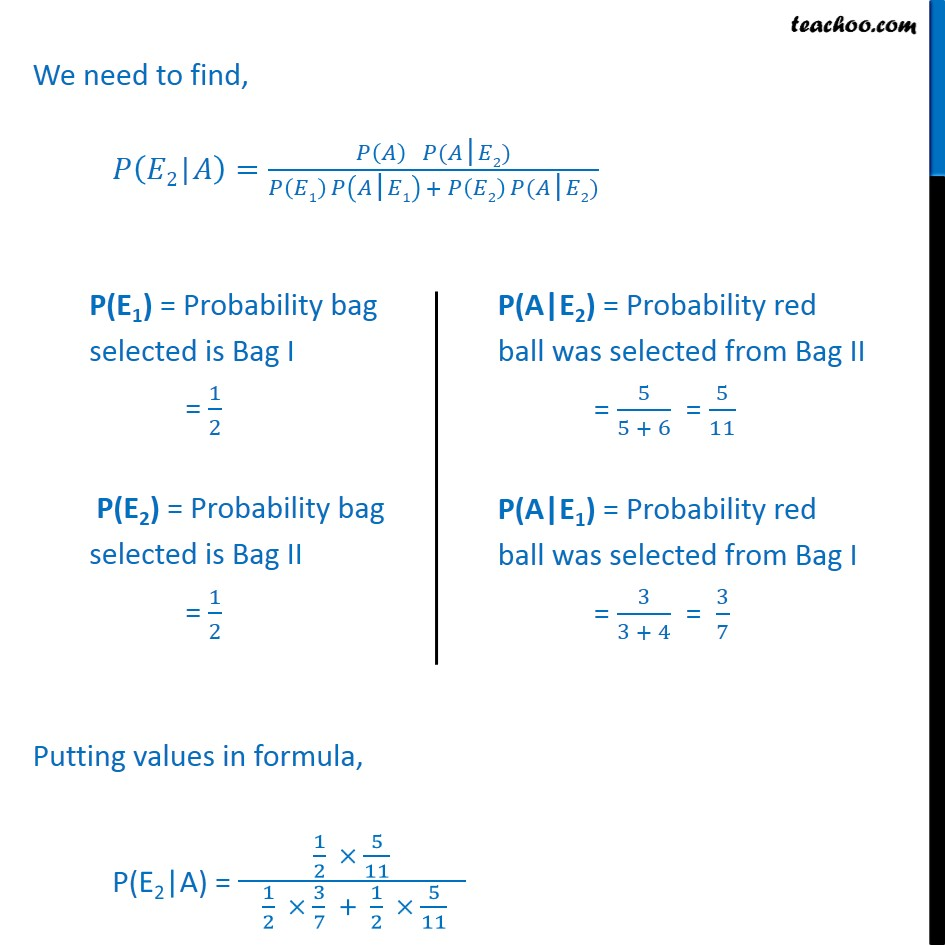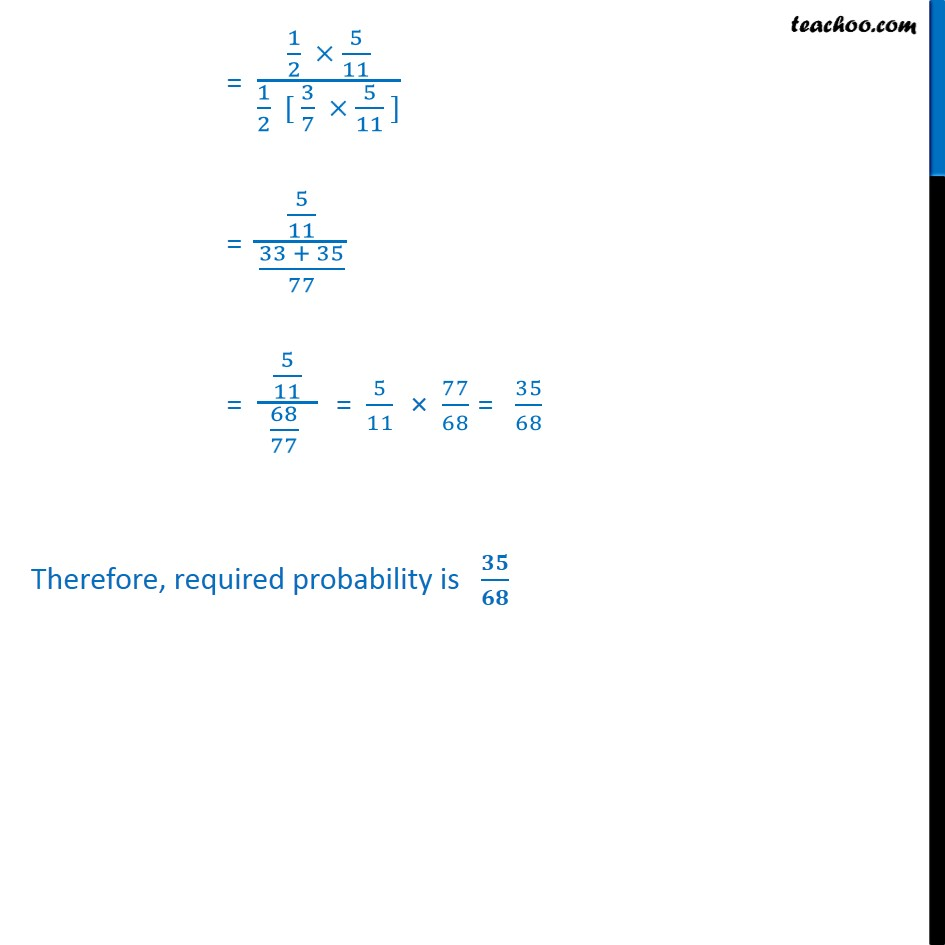1. Chapter 13 Class 12 Probability (Term 2)
2. Serial order wise
3. Examples

Transcript

Example 16 Bag I contains 3 red and 4 black balls while another Bag II contains 5 red and 6 black balls. One ball is drawn at random from one of the bags and it is found to be red. Find the probability that it was drawn from Bag II. Let E1 : Bag selected is bag 1 E2 : Bag selected is bag 2 A : Ball selected is Red B : Ball selected is Black We need to find P(ball was drawn from bag 2, if ball is red) = P(E2|A) We need to find, 𝑃 𝐸2|𝐴﷯= 𝑃 𝐴﷯ 𝑃(𝐴|𝐸2)﷮𝑃 𝐸1﷯ 𝑃 𝐴|𝐸1﷯ + 𝑃 𝐸2﷯ 𝑃(𝐴|𝐸2)﷯ Putting values in formula, P(E2|A) = 1﷮2﷯ × 5﷮11﷯﷮ 1﷮2﷯ × 3﷮7﷯ + 1﷮2﷯ × 5﷮11﷯ ﷯ = 1﷮2﷯ × 5﷮11﷯﷮ 1﷮2﷯ [ 3﷮7﷯ × 5﷮11﷯ ]﷯ = 5﷮11﷯﷮ 33 + 35﷮77﷯﷯ = 5﷮11﷯﷮ 68﷮77﷯ ﷯ = 5﷮11﷯ × 77﷮68﷯ = 35﷮68﷯ Therefore, required probability is 𝟑𝟓﷮𝟔𝟖﷯

Examples